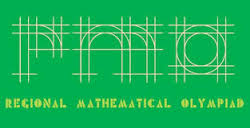# Indian Regional Mathematical Olympiad Problem 2

Algebra Level 5Let $f(x) = x^3 + ax^2 + bx + c$ and $g(x) = x^3 + bx^2 + cx + a$, where $a, b, c$ are integers with $c\neq 0$. Suppose that the following conditions hold: (a) $f(1) = 0$; (b) the roots of $g(x) = 0$ are the squares of the roots of$f(x) = 0$. Find the value of $a^{2013}+b^{2013}+c^{2013}$.

×